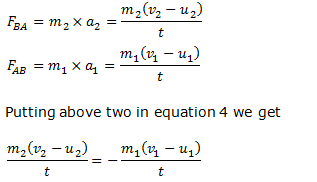# Law of conservation of momentum

## 8. Law of conservation of momentum

• Law of conservation of linear momentum is a extremely important consequence of Newton's third law of motion in combination with the second law of motion.
• According to law of conservation of momentum
When two or more bodies acts upon each other their total momentum remains constant provided no external forces are acting
• So, Momentum is never created or destroyed.
• When this law is applied for a collision between two bodies, the total momentum of the colliding bodies before collision is equal to the total momentum after collision.
• We can apply this law for a collision between two vehicles. This law is applicable for all types of collisions.
• Consider two particles say A and B of mass m1 and m2 collide with each other and forces acting on these particles are only the ones they exert on each other.
• Let u1 and v1 be the initial and final velocities of particle A and similarly, u2 and v2for particle B. Let the two particles be in contact for a time t. So, Change in momentum of A=m1 (v1-u1) Change in momentum of B=m2 (v2-u2 )

• During the collision, let A impart an average force equal to FBA on B and let B exert an average FAB on A. We know that from third law of motion FBA=-FAB            (4)
Here,canceling t on both sides and rearranging the equation we get
${m_1}{u_1} + {m_2}{u_2} = {m_1}{v_1} + {m_2}{v_2}$              (5)
Now, ${m_1}{u_1} + {m_2}{u_2}$ represents the total momentum of particles A and B before collision and ${m_1}{v_1} + {m_2}{v_2}$ represents the total momentum of particles after collision. This means that
Total momentum before collision=total momentum after collision
• Equation 5 which ${m_1}{u_1} + {m_2}{u_2} = {m_1}{v_1} + {m_2}{v_2}$ ,is known as the law of conservation of momentum.
• Thus we conclude that when two particles are subjected only to their mutual interactions ,the sum of the momentums of the bodies remains constant in time or we can say the total momentum of the two particles does not change because of the any mutual interactions between them.
• For any kind of force between two particles then sum of the momentum ,both before and after the action of force should be equal i.e total momentum remains constant.
• Law of conservation of linear momentum is one of the most fundamental and important principle of mechanics.
• Once again ,the total momentum of two or any number of particles of interacting particles is constant if they are isolated from outside influences (or no resultant external forces is acting on the particles).

Reference Books for class 9 science

Given below are the links of some of the reference books for class 9 science.

These books by S.Chand Publications are detailed in their content and are must have books for class 9 students. Some other books that might be worth considering are
1. Oswaal CBSE Question Bank Class 9 Science Chapterwise & Topicwise We all know importance of practicing what we have studied. This book is perfect for practicing what you have learned and studied in the subject.
2. Pearson Foundation Series (IIT-JEE/NEET) Physics, Chemistry, Maths & Biology for Class 9 (Main Books) | PCMB Combo These Foundation books would be helpful for students who want to prepare for JEE/NEET exams. Only buy them if you are up for some challenge and have time to study extra topics. These might be tough for you and you might need extra help in studying these books.
3. Foundation Science Physics for Class - 9 by H.C. Verma This is one of my favorite Physics book for class 9. Most of the book is within the limits of CBSE syllabus. It might overwhelm you with its language but I feel if you can understand the content is authentic with plenty of problems to solve.

You can use above books for extra knowledge and practicing different questions.

Note to our visitors :-

Thanks for visiting our website.